# Calculator Techniques for Mathematics Using Casio Calculators

• Author:
• Updated date:

Ray is a licensed engineer in the Philippines. He loves to write about mathematics and civil engineering.

Mathematics is one of the most illimitable problems in board examinations. It is very eclectic I must say. Mathematics is one of the subjects with the highest components in Engineering Board Examinations having 1/3 of the total percentage of the coverage and in some cases, more than that. In line with your primary disciplines, mathematics is one of the toughest parts in Engineering Board Examinations. It becomes difficult because subjects that you have taken in College for three or more semesters are concentrated. So, how do you aid this?

Mathematics should be the scoring section in your exam and requires the smallest amount of effort. Unfortunately, it turns out to be the opposite. Many are struggling to get high scores in the Mathematics section in the board exam. The proven reasons are the lack of techniques and intellectual strategies in solving difficult questions. That is why knowing the calculator techniques will hugely save your time and stamina, give you time to review your answers and let you achieve your desired score.

Topics Included Under Mathematics in Engineering Board Exams

• Algebra
• Trigonometry
• Plane and Solid Analytic Geometry
• Plane and Solid Mensuration
• Differential Calculus
• Integral Calculus
• Differential Equations
• Advanced Engineering Mathematics
• Engineering Economy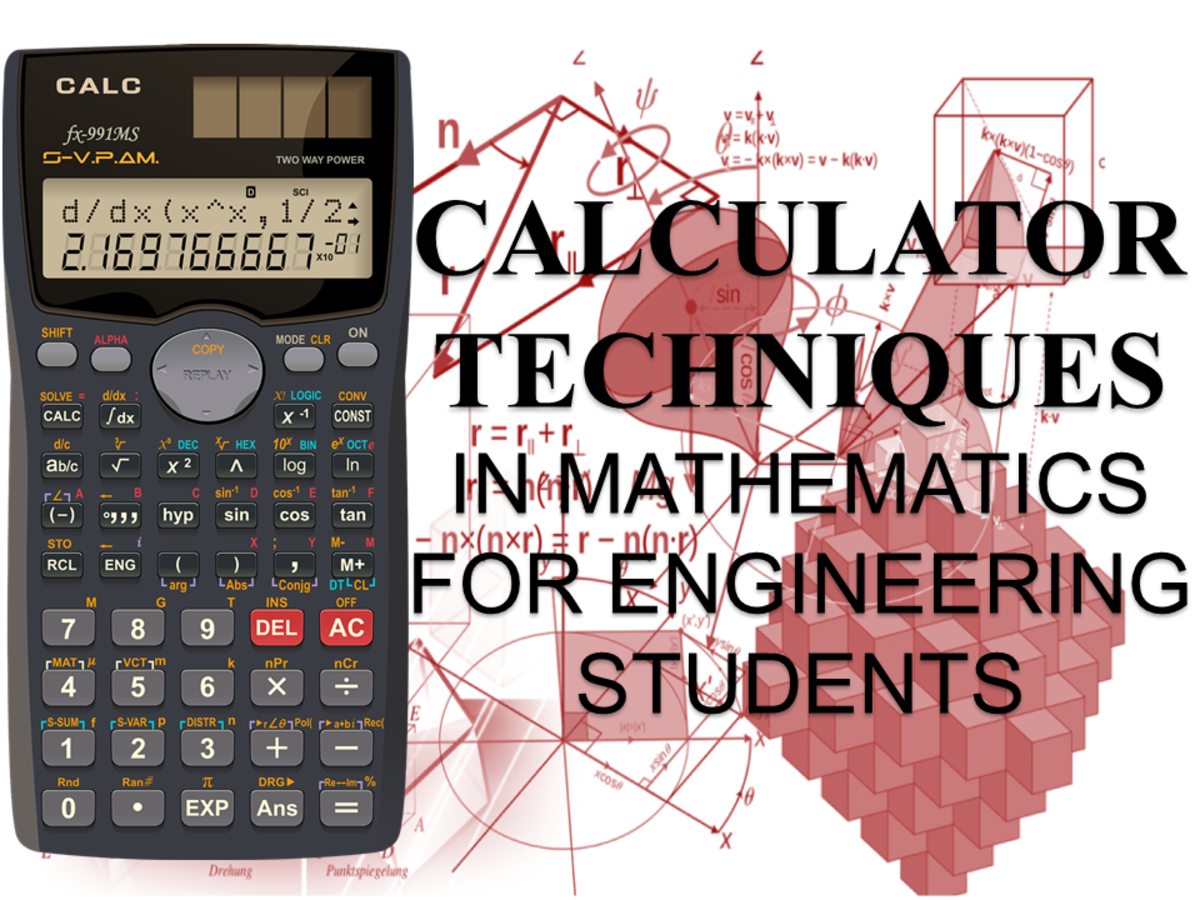Calculator Techniques in MathJohn Ray Cuevas

For every calculator technique solution, there is a conventional method of solving provided. If you have problems encountered with the Casio Calculator, refer to the user's manual for a complete list of functions. Here are the most valuable calculator techniques in mathematics that you should know. Note that these calculator features may not be available in low-quality calculators. I highly encourage you to use scientific calculators produced by large calculator companies like Casio and Sharp.

## Problem 1: Nth Term of an Arithmetic Progression

Find the 26th term of the arithmetic progression 5, 9, 13, 17, . .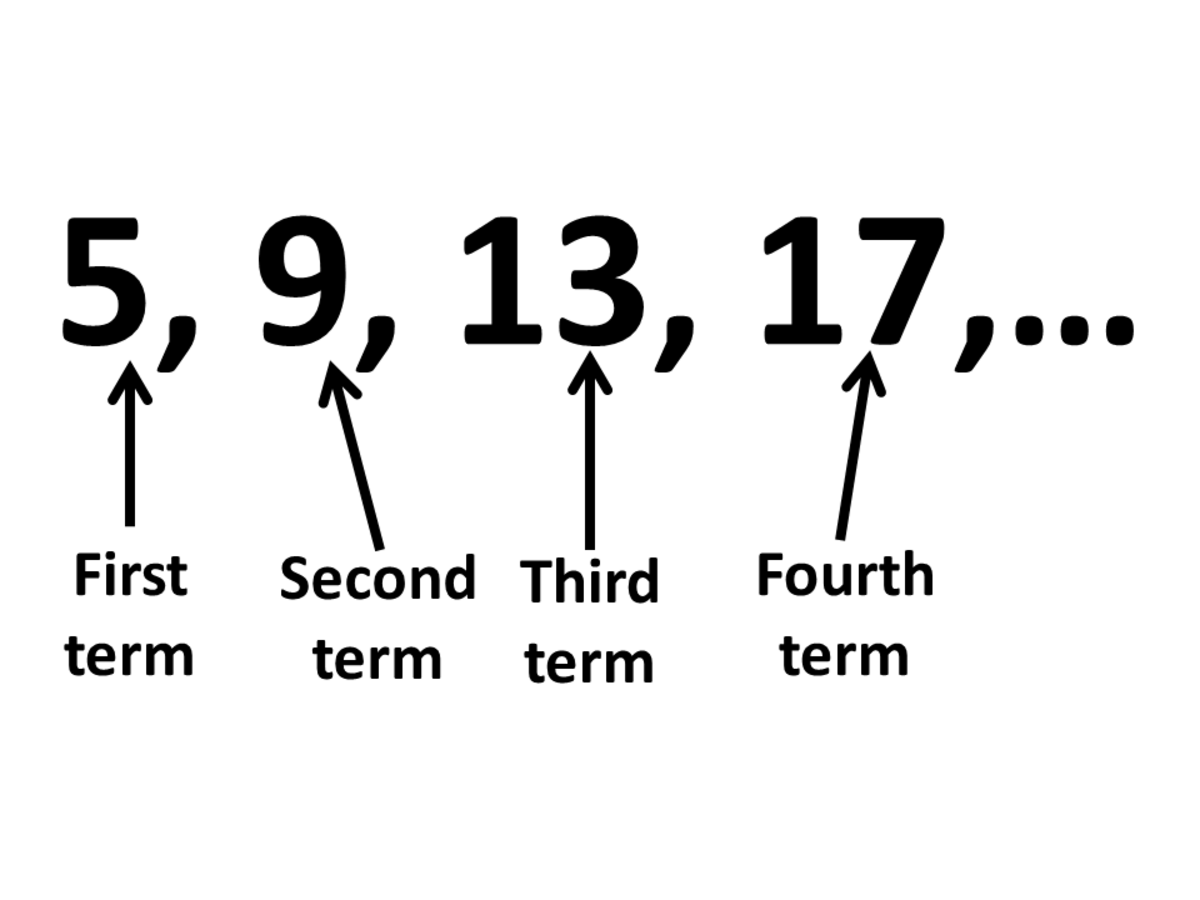Calculator Techniques in AlgebraJohn Ray Cuevas

### Solution 1: Conventional Solution

a. Using the formula provided below, compute for the 26th term manually by method of substitution.

an = a1 + (n - 1) d

a26 = 5 + (26 - 1) (4)

a26 = 105

Answer: The 26th term is 105.

### Solution 2: Calculator Technique

a. Solve for the answer using a calculator technique using linear regression. Simply follow the steps provided below.

Input these values.

XY

1

5

2

9

Answer: The 26th term is 105.

## Problem 2: Geometric Progression

In a geometric progression, the term 6th is 4096, and the 10th term is 1048576. What is the value of the 4th term?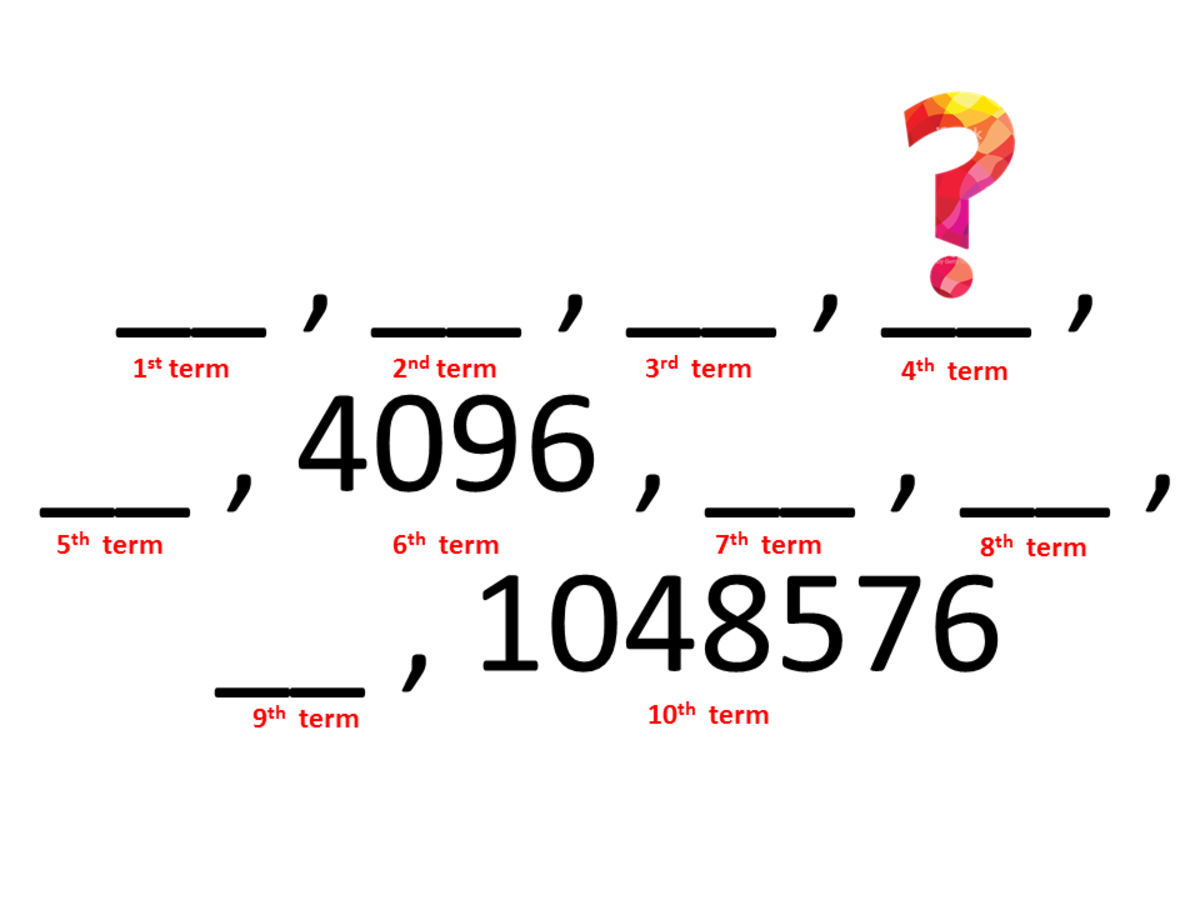Calculator Technique for Geometric ProgressionJohn Ray Cuevas

### Solution 1: Conventional Solution

a. The formula for geometric progression is an= a1 rn-1. From the given values, we can make 2 equations having 2 unknowns.

Scroll to Continue

Equation 1:

a6 = a1 r6-1

4096 = a1 r5

Equation 2:

a10 = a1 r10-1

1,048,576 = a1 r9

b. Using any method to solve two equations and two unknowns,solve for the values of a1 and r. It must result to these two answers.

a1 = 4

r = 4

c. Substitute the values obtained in the main equation.

a4 = a1 r4-1

a4 = 4 (4)3

a4 = 256

Answer: The 4th term is 256.

### Solution 2: Calculator Technique

a. Solve for the answer using a calculator technique using regression. Simply follow the steps provided below.

Input values

XY

6

4096

10

1,048,576

Answer: The 4th term is 256.

## Problem 3: Sum of a Sequence

How many terms of the sequence 2, 4, 6... must be taken so that the sum is 3660?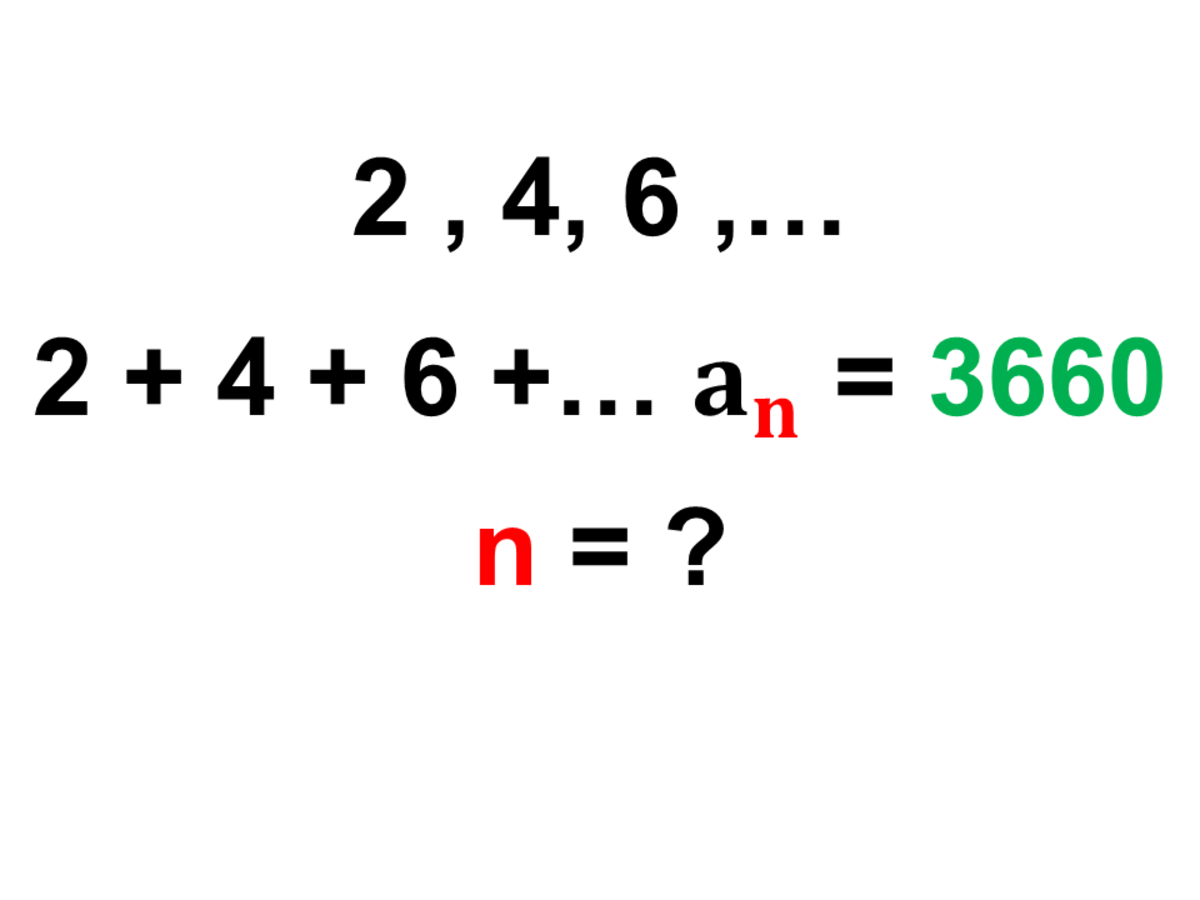Calculator Techniques for AlgebraJohn Ray Cuevas

### Solution 1: Conventional Solution

a. Given the following values, substitute them in the given equation below and solve for n.

Sn = 3660

a1 = 2

Sn = (n/2)[2a1+(n-1)(d)]

3660 = (n/2)[2(2)+(n-1)(2)]

n = 60

Answer: The number "n" terms to get a sum of 3660 is 60.

### Solution 2: Calculator Technique

a. Follow the steps below in order to solve the problem using the calculator technique.

Input values

XY

0

0

1

2

2

2+4

Answer: The number "n" terms to get a sum of 3660 is 60.

## Problem 4: Hand-Clock Problems

At what time between 2 PM till 3 PM will the hands of the clock be at the right angle?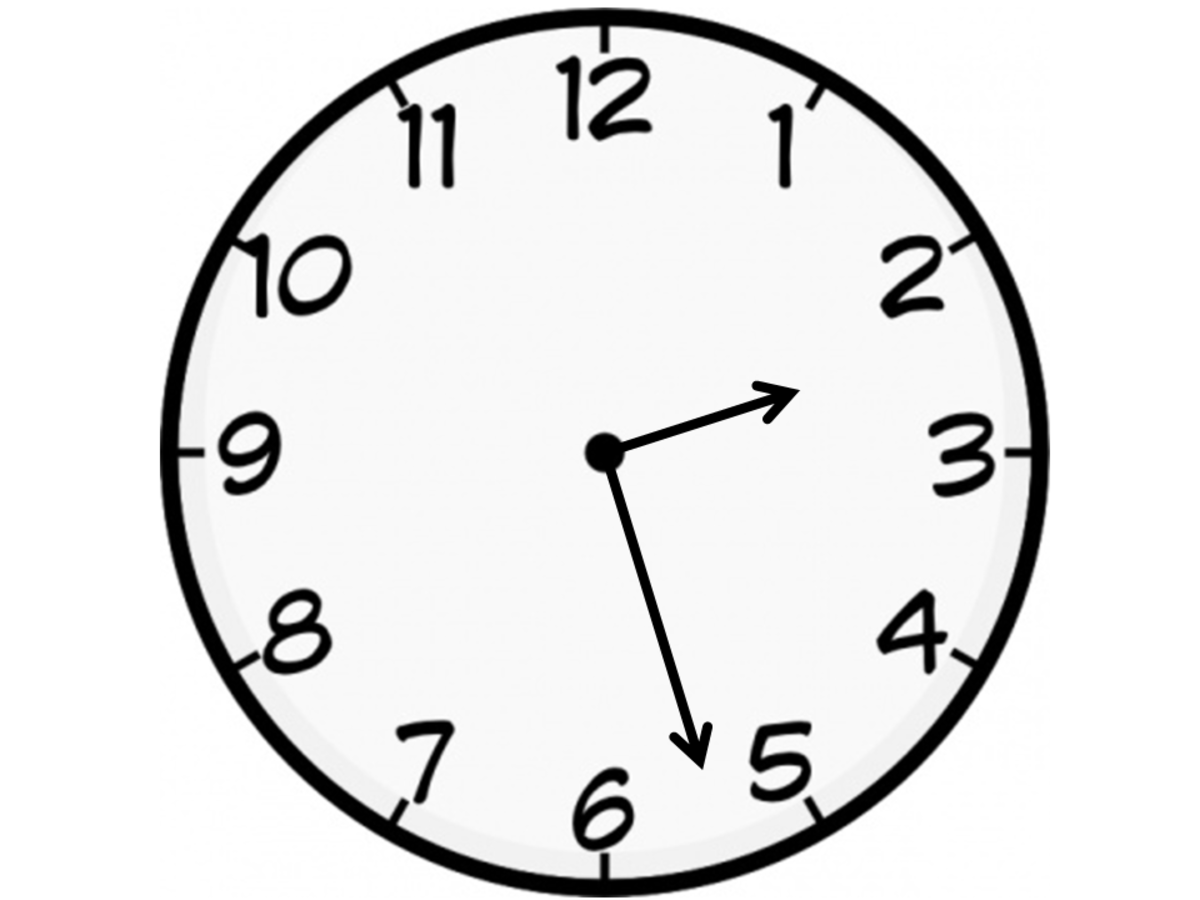Calculator Techniques for Hand Clock ProblemsJohn Ray Cuevas

### Solution 1: Calculator Technique

1. Press "MODE" button.

2. Choose "3: STAT".

3. Choose "2: A+BX".

Under the X column, input the two values of time. Take note that -30 and 330 here is constant. Multiply -30 by the first time. Then, add the product to 330.

XY

2

-30(2)

3

330 + [-30(2)]

4. Press AC.

5. SHIFT-STAT.

6. Choose "5: Reg".

7. Choose "4: x".

8. Input 90 beside x. It should look like this 90x. Press Enter.

Answer: The resulting answer should be 2:27:16.36 PM.

## Problem 5: Hand-Clock Problems

What is the angle between the hands of the clock at 2:35:16 in radians?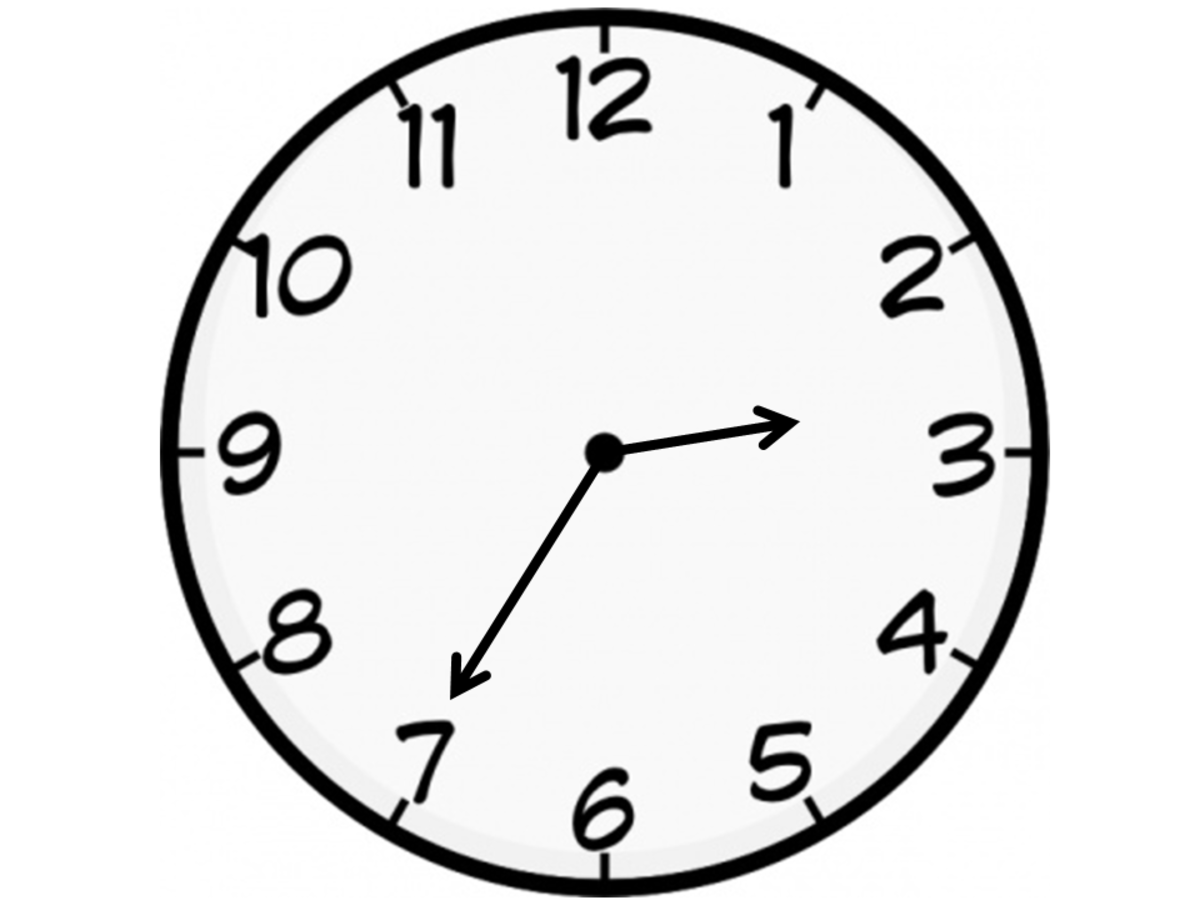Calculator Techniques for Hand Clock ProblemsJohn Ray Cuevas

### Solution 1: Calculator Technique

1. Press MODE.

2. Choose "3: STAT".

3. Choose "2: A+BX".

XY

2

-30(2)

3

330 + (-60)

4. Press AC.

5. SHIFT-STAT.

6. Choose "5: Reg".

7. Choose "5: ŷ".

8. Input 2:35:16 beside ŷ. Press Enter. The answer should be 133.97 deg. Convert it to radians. The final answer is 2.338 radians.

## Problem 6: Two Unknown Sides of a Triangle

A triangle has a base of 10 m and angles 35° and 64° respectively. Find the length of the other two sides.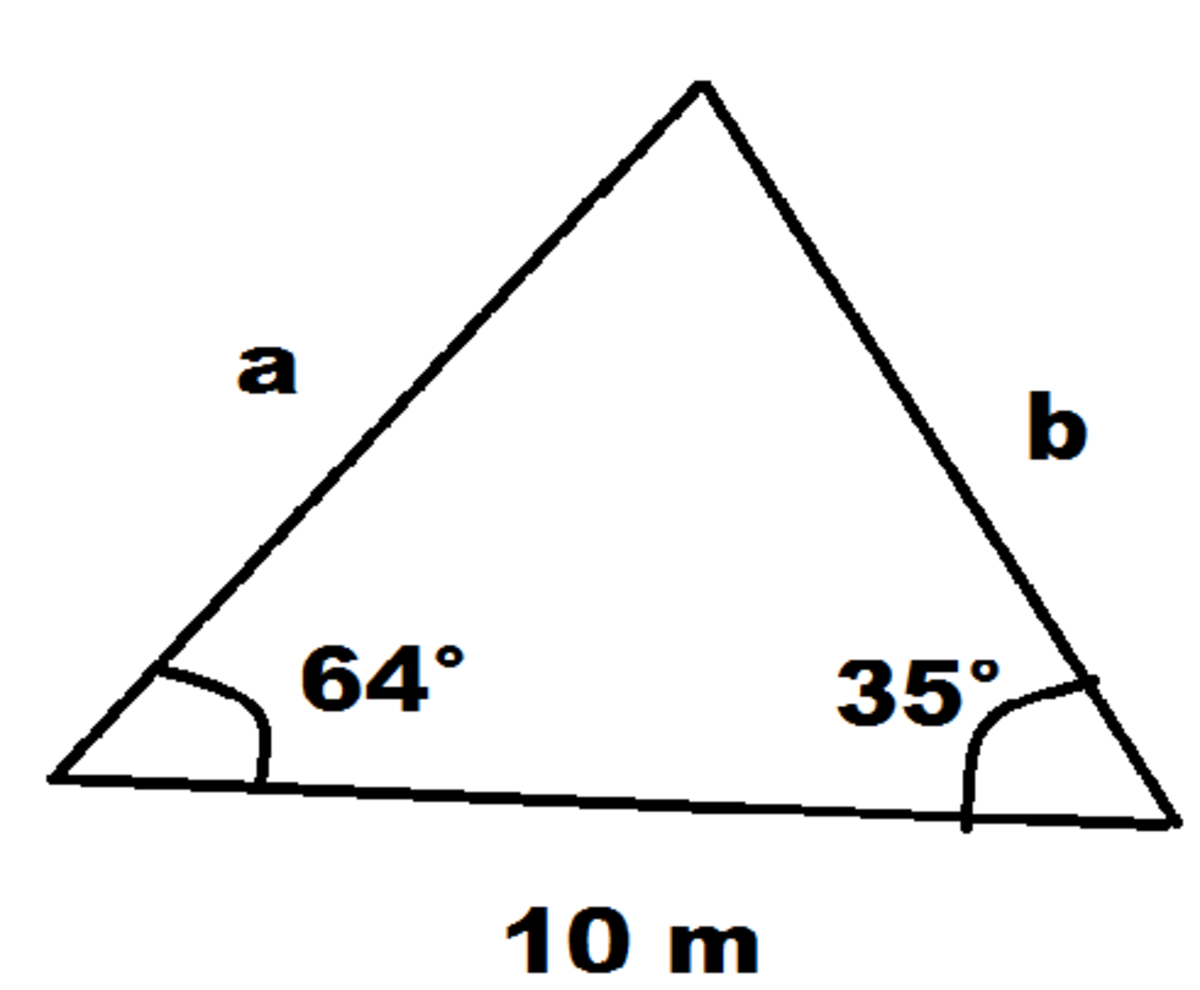Calculator Technique for Unknown Sides of a TriangleJohn Ray Cuevas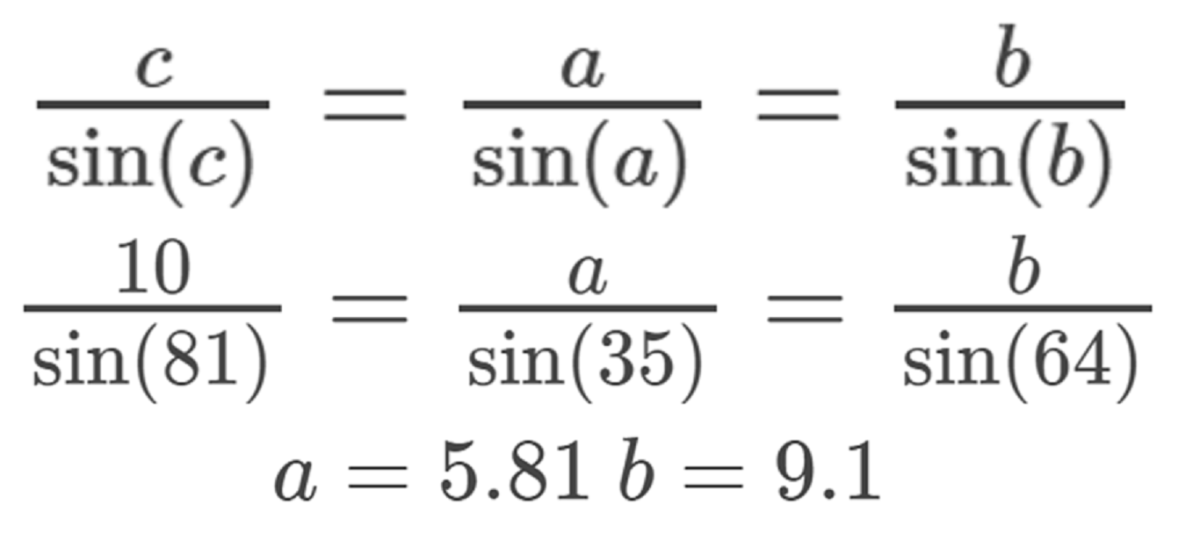Solution 1: Conventional SolutionJohn Ray Cuevas

### Solution 2: Calculator Technique

1. Press MODE.

2. Choose "5: EQN".

3. Choose "1: anX + bnY = cn".

After putting these values, press the Enter button and you'll get the same answer.

axbyc

cos(64)

cos(35)

10

sin(64)

-sin(35)

0

Answer: The value of a is 5.81 and the value of b is 9.1.

## Problem 7: Three Circles Tangent to Each Other

Three circles are mutually tangent to one another externally. Connecting the 3 centers form a triangle whose sides are 16 cm, 20 cm, and 24 cm. What is the area of the smallest circle in cm2?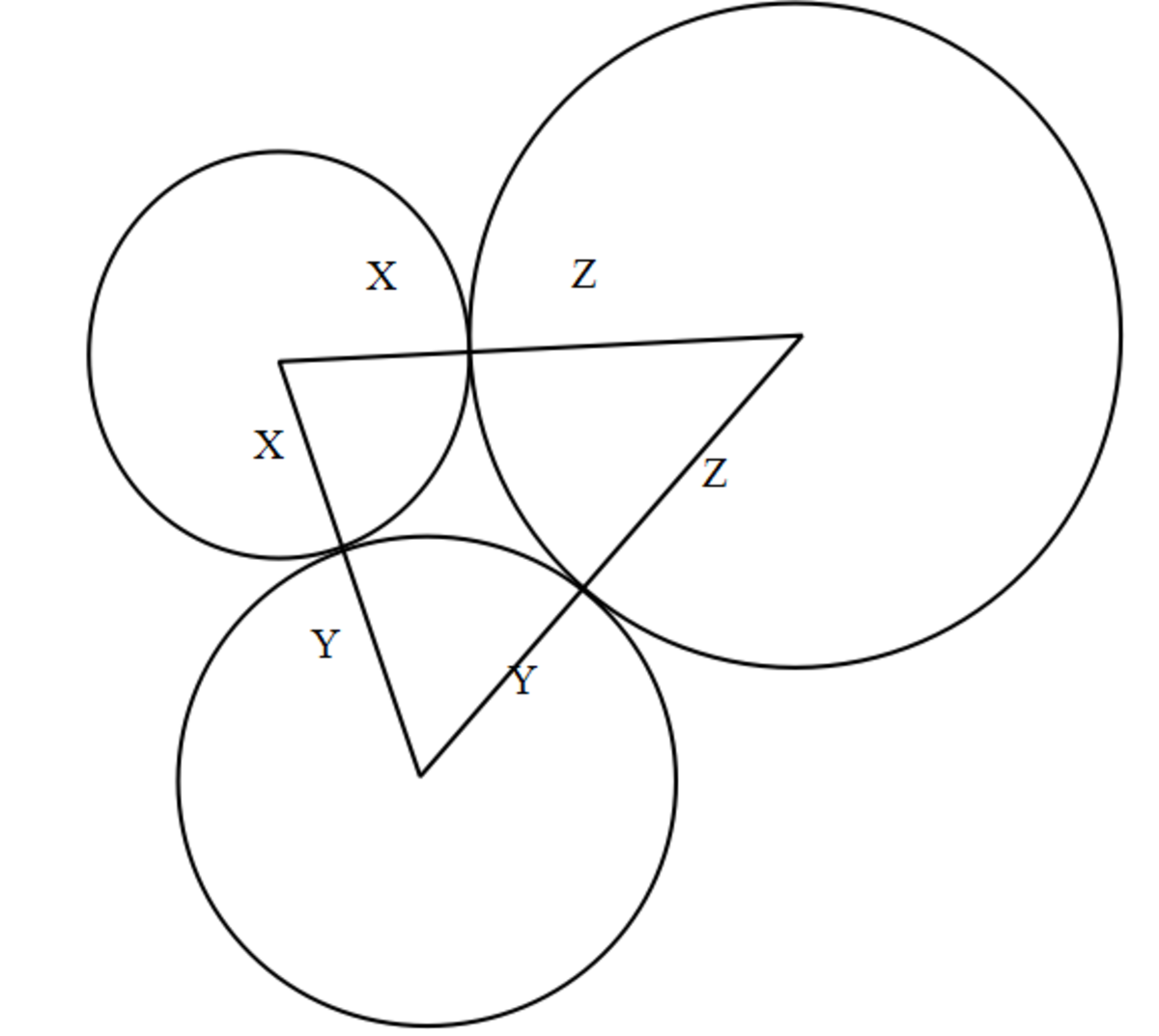Calculator Techniques for Circles Tangent to Each OtherJohn Ray Cuevas

### Solution 1: Conventional Solution

X + Y = 16 ← eq. 1

X + Z = 20 ← eq. 2

Y + Z = 24 ← eq. 3

a. Solving manually, you'll arrive with the answers.

X = 6 cm

Y = 10 cm

Z = 14 cm

### Solution 2: Calculator Technique

1. Press MODE.

2. Choose "5: EQN".

3. Choose "2: anX + bnY + cnZ= dn".

 1 1 0 16 1 0 1 20 0 1 1 24

Answer: The final value of x is 6 centimeters, y is 10 centimeters, and z is 14 centimeters.

## Problem 8: Equation of a Parabola

A parabola has its axis parallel to the y-axis and passes through (4, 5), (-2, 11) and (-4, 21). What is the equation of the parabola?

### Solution 1: Calculator Technique

1. Press MODE.

2. Choose "3: STAT".

3. Choose "3: ±cX2".

XY

4

5

-2

11

-4

21

4. Press "AC".

5. Press "SHIFT 1 5 1". Store at A.

6. Press "SHIFT 1 5 2". Store at B

7. Press "SHIFT 1 5 3". Store at C.

The final answer will be in the form A+BX+CX2.

Answer: The final answer is 5 - 2x + 0.5x2.

## Problem 9: Applications of Parabola

When the load is uniformly distributed horizontally, the cable of a suspension bridge hangs in a parabolic arc. If the bridge is 300 ft long, the tower is 60 ft. high, and the cable is 20 ft. above the roadbed at the center, find the distance from the roadbed 50 ft. from the center.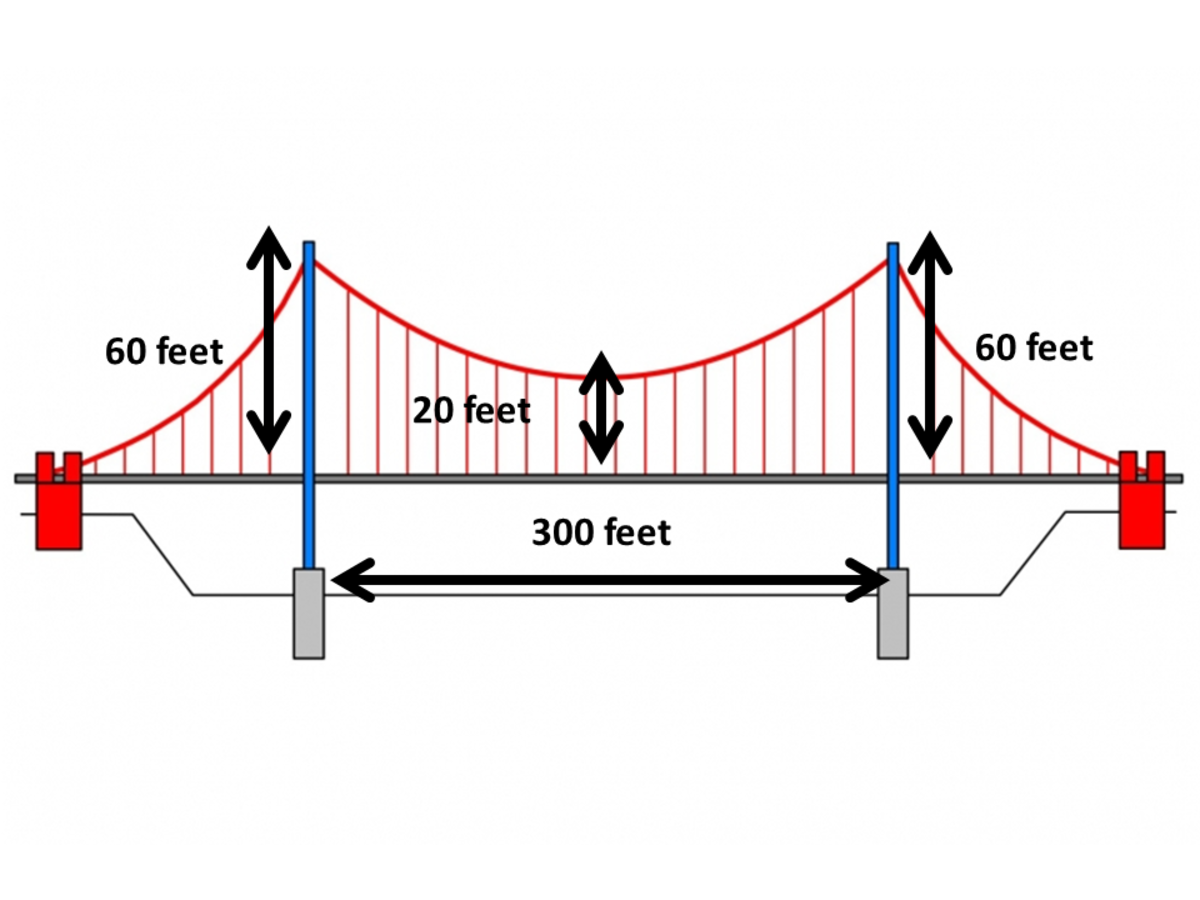Calculator Techniques for ParabolaJohn Ray Cuevas

### Solution 1: Calculator Technique

1. Press the "MODE" button.

2. Choose "3: STAT".

3. Choose "3: ±cX2".

XY

-150

60

0

20

150

60

4. Press "AC".

5. SHIFT-STAT

6. Choose "5: Reg".

7. Choose "6: y". Place 50 beside y.

## Problem 10: Volume of Water in a Sphere

A sphere of radius 15 cm. contains water at a height of 24 cm. Find the volume of water inside the sphere.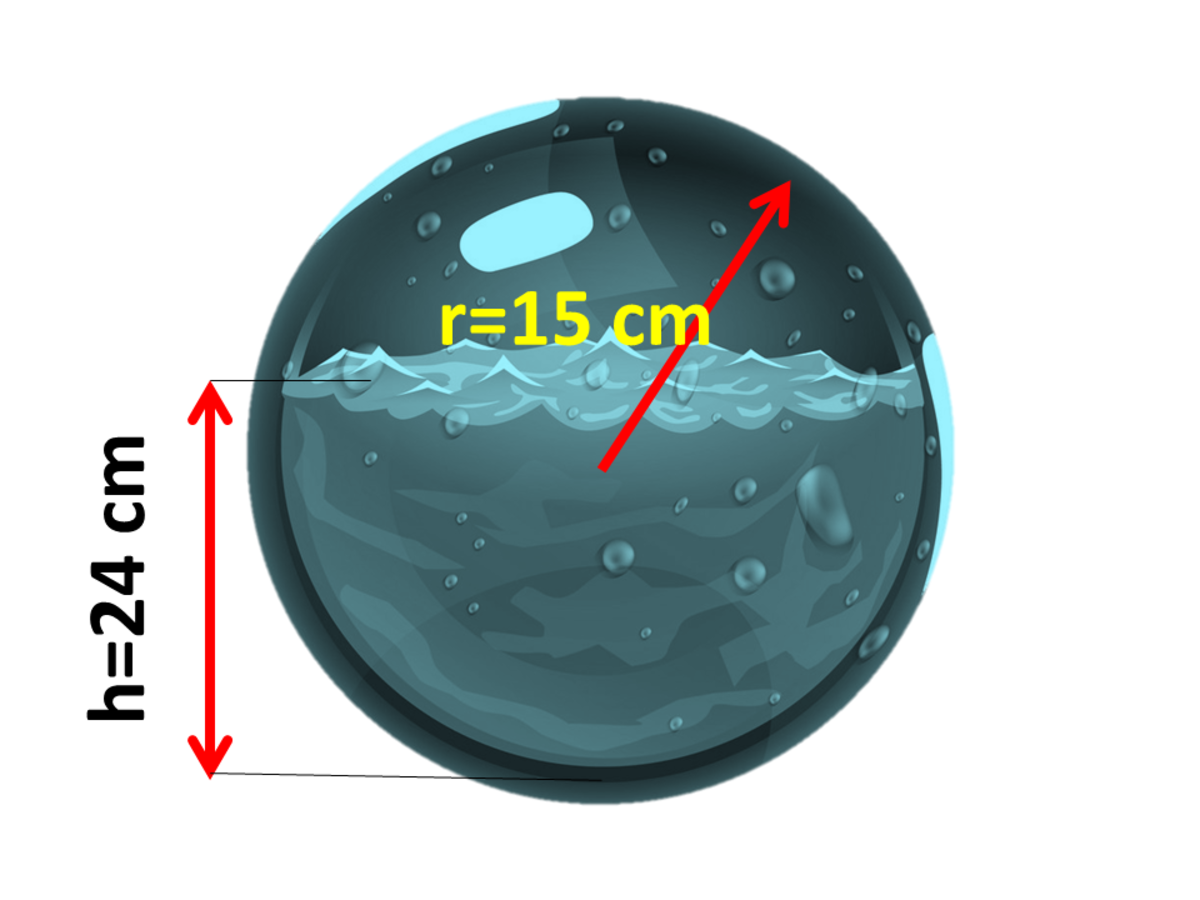Calculator Techniques for Solid GeometryJohn Ray Cuevas

### Solution 1: Calculator Technique

1. Press the "MODE" button.

2. Choose "3: STAT".

3. Choose "3: ±cX2".

XY

0

0

15

pi(15)^2

30

0

4. Press "AC".

5. Press "SHIFT 1 5 1". Store at A.

6. Press "SHIFT 1 5 2". Store at B

7. Press "SHIFT 1 5 3". Store at C.

8. Go back to mode 1.

9. Get the integral in a form A+BX+CX2. Evaluate from 0 to 24.

Answer: The final volume of water is V = 4032π cm3.

## Problem 11: Evaluating Limits

Evaluate the limit of (x2 + x -12)/(2x2 - 7x +3) as x approaches 3.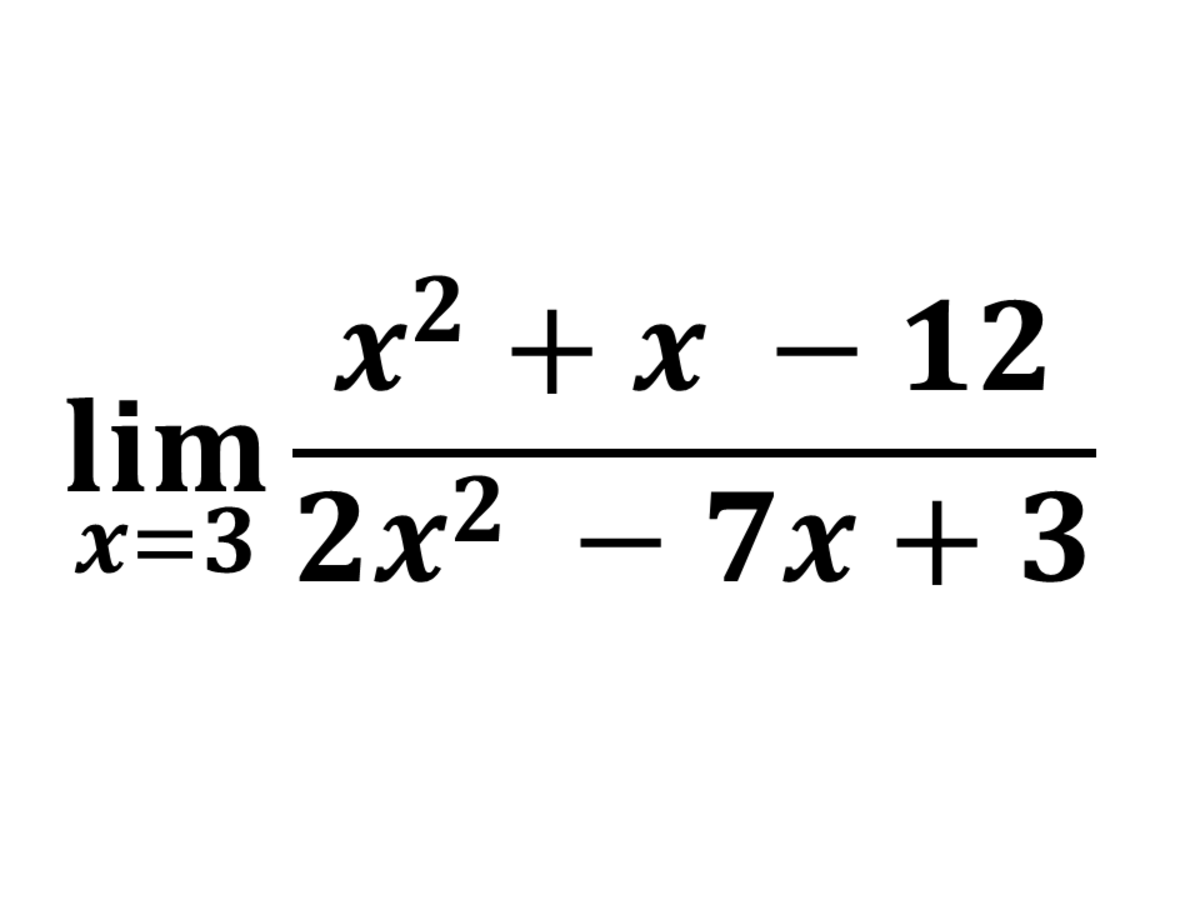Calculator Techniques for CalculusJohn Ray Cuevas

### Solution 1: Calculator Technique

1. Input the given equation into your calculator.

2. Press "CALC".

3. Input 3.000001 or 2.999999

## Problem 12: Compound Interest

At what interest rate, compounded quarterly, will an investment double in 5 years?

### Solution 1: Calculator Technique

1. Assume money = Y = 1.

2. Press "MODE" button.

3. Choose "3: STAT".

4. Choose "A*B^X".

XY

0

1

20

2

5. Press "AC" button.

6. Press "SHIFT 1 5 2".

7. Subtract the result by 1 and multiply by 4 (5-1).

Answer: The interest rate is 14.05 %.

## I'll Add More Calculator Techniques

This content is accurate and true to the best of the author’s knowledge and is not meant to substitute for formal and individualized advice from a qualified professional.